# Presetting#

Awesome presets, built-in!

## preset keywords#

ahlive has these keywords for presetting data:

ahlive keyword

charts

description

trail

scatter

have a line or point follow the original

race

bar, barh

move bars around by ranking

delta

bar, barh

stacked

bar, barh

pile bars atop rather than side-by-side

morph

line, scatter, bar, barh, errorbar, area

plot all states simultaneously

morph_trail

line, scatter, errorbar, area

plot all states simultaneously plus trail

morph_stacked

bar, barh

plot all states simultaneously plus stacked

rotate

line, scatter, errorbar, area, pcolormesh, contourf, contour, hexbin, quiver, streamplot

show data on a revolving globe

scan_x

pcolormesh, contourf, contour, hexbin, quiver, streamplot

replace old data with new from left to right

scan_y

pcolormesh, contourf, contour, hexbin, quiver, streamplot

replace old data with new from top to bottom

## trail#

trail allows scatter charts to leave behind footprints.

:

import ahlive as ah
ah.Array([0, 1, 2], [3, 4, 5], color="red", preset='trail').render()

gifsicle: warning: trivial adaptive palette (only 210 colors in source)

: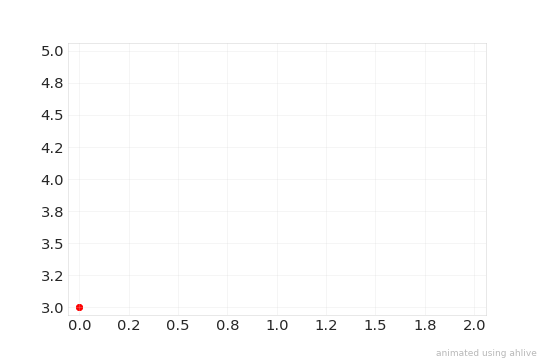trail can be configured to expire in number of base states and only show a trailing line.

:

import ahlive as ah
arr = ah.Array([0, 1, 2], [0, 1, 0], chart='scatter', preset='trail')
arr.config('preset', chart='line', expire=1).render()

: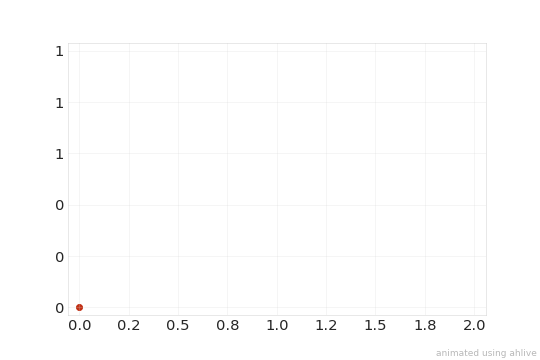## race#

race will yield bar chart races as long as there is more than one item overlaid. It’s also possible to customize bar_label and ascending.

:

import ahlive as ah
import pandas as pd
x = ['a', 'a', 'b', 'b']
y =  [4, 5, 3, 6]
df = pd.DataFrame({'x': x, 'y': y})
ah.DataFrame(
df, 'x', 'y', label='x', inline_labels='y',
chart='bar', preset='race'
).config(
'preset', bar_label=False, ascending=True
).render()

: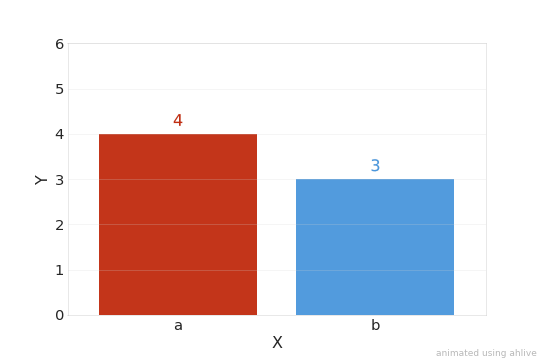## delta#

delta will highlight the differences in values for bar charts.

:

import ahlive as ah
import pandas as pd
x = ['a', 'a', 'b', 'b']
y =  [4, 5, 3, 8]
df = pd.DataFrame({'x': x, 'y': y})
ah.DataFrame(
df, 'x', 'y', label='x', inline_labels='y',
chart='barh', preset='delta'
).render()

: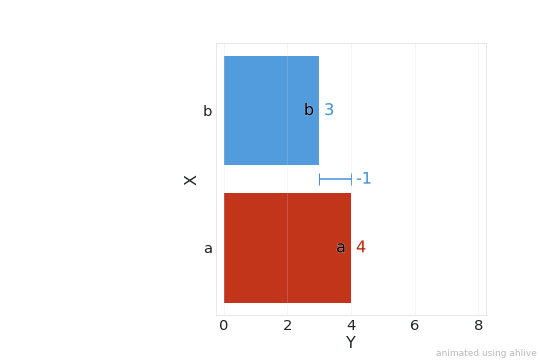## stacked#

Create stacked bar charts instead of the default side by side.

:

import ahlive as ah

x = ["X", "X"]
y1 = [1, 0]
y2 = [2, 0]

(ah.Array(x, y1, label="A", preset="stacked", chart="bar", revert="boomerang") *
ah.Array(x, y2, label="B", preset="stacked", chart="bar", ylims=(0, 3))
).render()

/home/docs/checkouts/readthedocs.org/user_builds/ahlive/conda/main/lib/python3.9/site-packages/ahlive-1.0.0.post14+dirty-py3.9.egg/ahlive/data.py:426: UserWarning: Multiple items found, you may want to use the 'morph' preset

: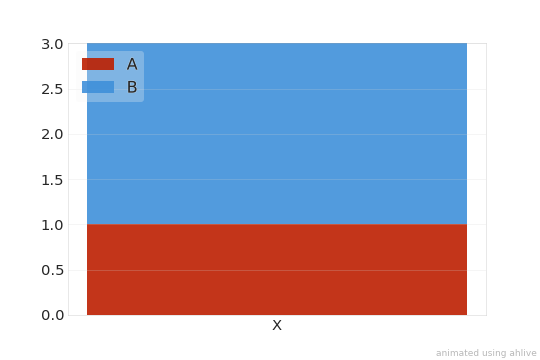## morph#

The initial states are batched together and each item gets morphed into a new state, grouped by group as the new items.

:

import ahlive as ah

x = [0, 1, 2, 3]
y1 = [4, 5, 6, 7]
y2 = [2, 10, 15, 20]

(
ah.Array(x, y1, group="A") *  # item 1, state 1
ah.Array(x, y2, group="A") *  # item 1, state 2
ah.Array(x, y2, group="B") *  # item 2, state 1
ah.Array(x, y1, group="B", chart="line", preset="morph", fps=30, revert="boomerang")  # item 2, state 2
).render()

: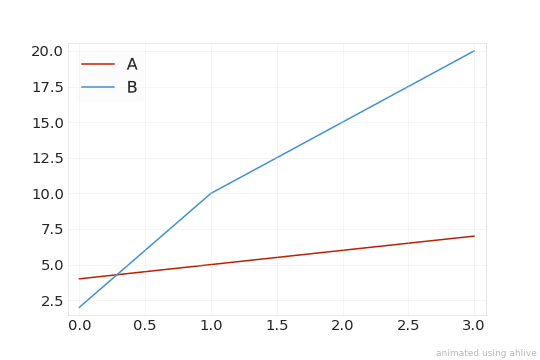## morph_trail#

Same as morph with a footprint.

:

import ahlive as ah

x = [0, 1, 2]
y1 = [0, 36, 0]
y2 = [0, 72, 0]
y3 = [0, 144, 0]

(
ah.Array(x, y1, group="a") *
ah.Array(x, y3, group="a") *
ah.Array(x, y2, group="b") *
ah.Array(x, y1, group="b", preset="morph_trail", chart="line", revert="boomerang")
).config(
"preset", color="gray"
).render()

: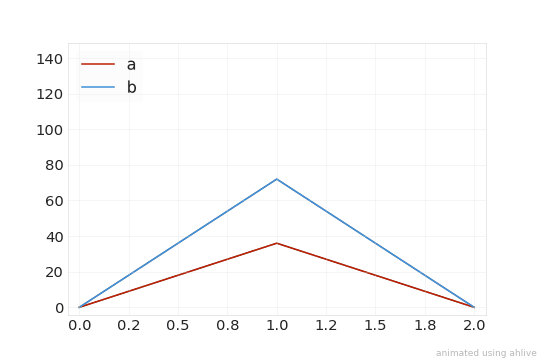## morph_stacked#

Same as morph, but for bar and barh charts: stacking separate groups.

:

import ahlive as ah

x = [0, 1]
y1 = [0, 0.8]
y2 = [1, 0.2]

(
ah.Array(x, y1, group="A") *  # item 1, state 1
ah.Array(x, y2, group="A") *  # item 1, state 2
ah.Array(x, y2, group="B") *  # item 2, state 1
ah.Array(x, y1, group="B", preset="morph_stacked", ylims="fixed",
fps=30, revert="boomerang", chart="barh")  # item 2, state 2
).render()

: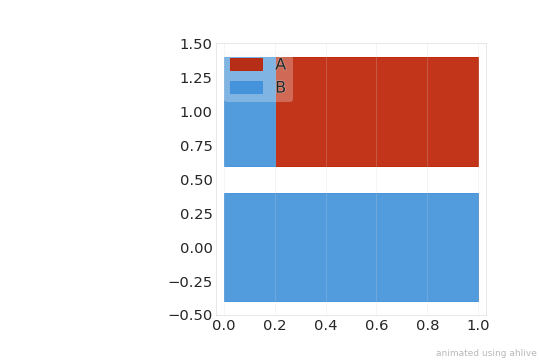## rotate#

rotate will yie ld a rotating globe for gridded charts. Geostationary views of the world can be achieved through worldwide=True, and the start and end of the central longitudes can be specified as a tuple in central_lon.

:

import xarray as xr
import ahlive as ah
ds = xr.tutorial.open_dataset('air_temperature').isel(time=slice(0, 8))
ah.Dataset(
ds, 'lon', 'lat', 'air', crs='platecarree', preset='rotate',
central_lon=(-90, -110), worldwide=True
).render()

/home/docs/checkouts/readthedocs.org/user_builds/ahlive/conda/main/lib/python3.9/site-packages/cartopy/io/__init__.py:241: DownloadWarning: Downloading: https://naturalearth.s3.amazonaws.com/110m_physical/ne_110m_coastline.zip

: